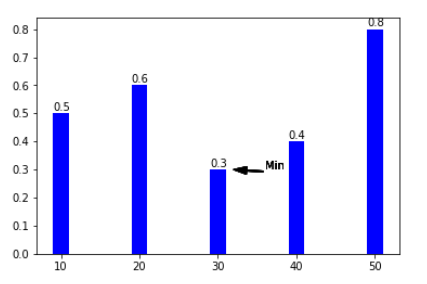# 介绍

Matplotlib 是支持 Python 语言的开源绘图库，因为其支持丰富的绘图类型、简单的绘图方式以及完善的接口文档，深受 Python 工程师、科研学者、数据工程师等各类人士的喜欢

Matplotlib 拥有着十分活跃的社区以及稳定的版本迭代，当我们在学习机器学习的课程时，掌握 Matplotlib 的使用无疑是最重要的准备工作之一

# 简单图形绘制

## 快速上手

``````import  matplotlib.pyplot as plt

%matplotlib inline

plt.plot([1, 2, 3, 2, 1, 2, 3, 4, 5, 6, 5, 4, 3, 2, 1])
``````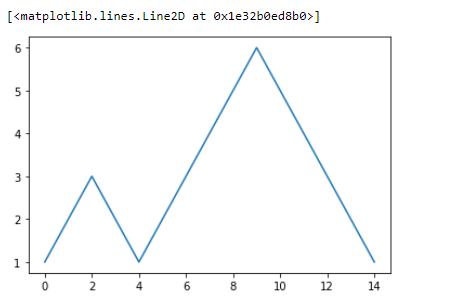`pyplot` 模块是 Matplotlib 最核心的模块，几乎所有样式的 2D 图形都是经过该模块绘制出来的

## 自定义X/Y轴

`plt.plot()``pyplot` 模块下面的直线绘制（折线图）方法类. 示例中包含了一个 `[1, 2, 3, 2, 1, 2, 3, 4, 5, 6, 5, 4, 3, 2, 1]` 列表，Matplotlib 会默认将该列表作为 y 值，而 x 值会从 0 开始依次递增。

``````plt.plot([1,2,3],
[1, 2, 3])
``````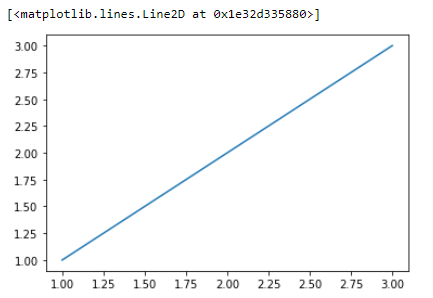# 图表实现

## 汇总

`pyplot` 模块中 `pyplot.plot` 方法是用来绘制折线图的

`matplotlib.pyplot.plot` 折线图
`matplotlib.pyplot.angle_spectrum` 电子波谱图
`matplotlib.pyplot.bar` 柱状图
`matplotlib.pyplot.barh` 直方图
`matplotlib.pyplot.broken_barh` 水平直方图
`matplotlib.pyplot.contour` 等高线图
`matplotlib.pyplot.errorbar` 误差线
`matplotlib.pyplot.hexbin` 六边形图案
`matplotlib.pyplot.hist` 柱形图
`matplotlib.pyplot.hist2d` 水平柱状图
`matplotlib.pyplot.pie` 饼状图
`matplotlib.pyplot.quiver` 量场图
`matplotlib.pyplot.scatter` 散点图
`matplotlib.pyplot.specgram` 光谱图

## 正弦曲线图

`matplotlib.pyplot.plot(*args, **kwargs)` 方法严格来讲可以绘制线形图或者样本标记

``````import numpy as np

# 在 -2PI 和 2PI 之间等间距生成 1000 个值，也就是 X 坐标
X = np.linspace(-2*np.pi, 2*np.pi, 1000)
# 计算 y 坐标
y = np.sin(X)

# 向方法中 `*args` 输入 X，y 坐标
plt.plot(X, y)
``````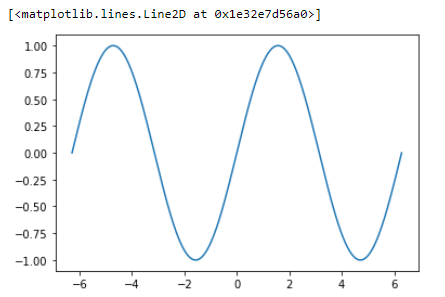## 柱状图

``````plt.bar([1,2,3],[1,2,3])
``````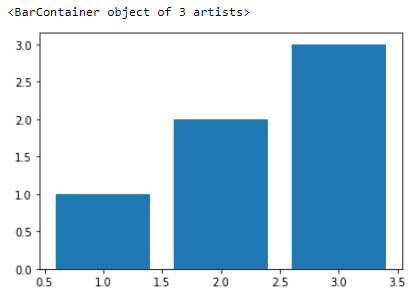## 散点图

``````# X,y 的坐标均有 numpy 在 0 到 1 中随机生成 1000 个值
X = np.random.ranf(1000)
y = np.random.ranf(1000)
# 向方法中 `*args` 输入 X，y 坐标
plt.scatter(X, y)
``````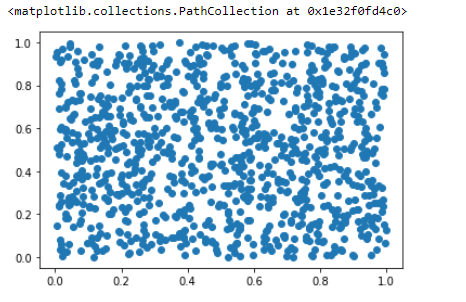## 饼图

``````plt.pie([1, 2, 3, 4, 5])
``````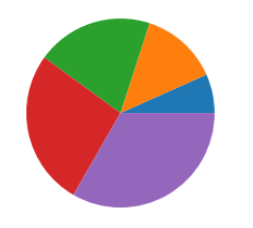## 量场图

``````X, y = np.mgrid[0:10, 0:10]
plt.quiver(X, y)
``````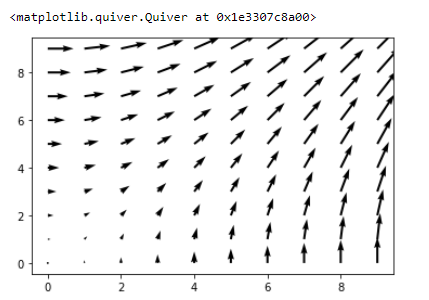## 等高线图

``````# 生成网格矩阵
x = np.linspace(-5, 5, 500)
y = np.linspace(-5, 5, 500)
X, Y = np.meshgrid(x, y)
# 等高线计算公式
Z = (1 - X / 2 + X ** 3 + Y ** 4) * np.exp(-X ** 2 - Y ** 2)

plt.contourf(X, Y, Z)
``````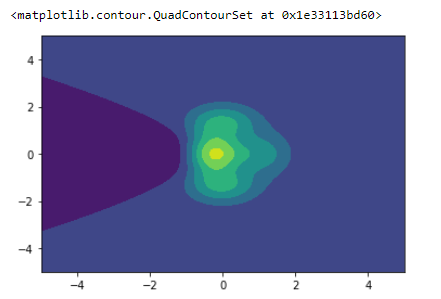# 图形样式

## 折线图

`alpha=` 设置线型的透明度，从 0.0 到 1.0
`color=` 设置线型的颜色
`fillstyle=` 设置线型的填充样式
`linestyle=` 设置线型的样式
`linewidth=` 设置线型的宽度
`marker=` 设置标记点的样式
…… ……

``````# 在 -2PI 和 2PI 之间等间距生成 1000 个值，也就是 X 坐标
X = np.linspace(-2 * np.pi, 2 * np.pi, 1000)
# 计算 sin() 对应的纵坐标
y1 = np.sin(X)
# 计算 cos() 对应的纵坐标
y2 = np.cos(X)

# 向方法中 `*args` 输入 X，y 坐标
plt.plot(X, y1, color='r', linestyle='--', linewidth=2, alpha=0.8)
plt.plot(X, y2, color='b', linestyle='-', linewidth=2)
``````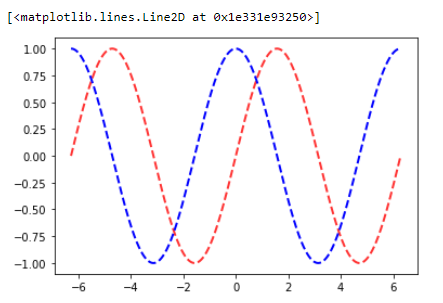## 散点图

`s=` 散点大小
`c=` 散点颜色
`marker=` 散点样式
`cmap=` 定义多类别散点的颜色
`alpha=` 点的透明度
`edgecolors=` 散点边缘颜色
``````# 生成随机数据
x = np.random.rand(1000)
y = np.random.rand(1000)
colors = np.random.rand(1000)
size = np.random.normal(50, 60, 1000)
# 绘制散点图
plt.scatter(x, y, s=size, c=colors)
``````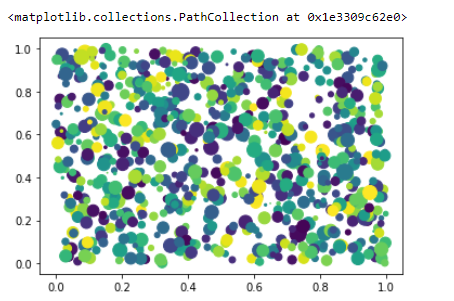## 饼图

``````# 各类别标签
label = 'a','b','c','d'
# 各类别颜色
color = 'r', 'g', 'r', 'g'
# 各类别占比
size = [1, 2, 3, 4]
# 各类别的偏移半径
explode = (0, 0, 0, 0, 0.2)
# 绘制饼状图
plt.pie(size, colors=color, explode=explode,
# 饼状图呈正圆
plt.axis('equal')
``````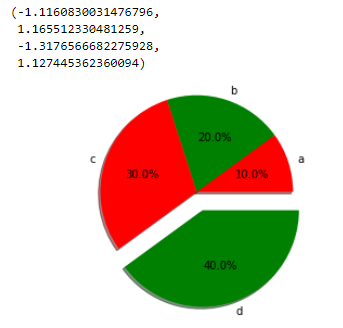# 组合图形样式

``````x = [1, 3, 5, 7, 9, 11, 13, 15, 17, 19]
y_bar = [3, 4, 6, 8, 9, 10, 9, 11, 7, 8]
y_line = [2, 3, 5, 7, 8, 9, 8, 10, 6, 7]

plt.bar(x, y_bar)
plt.plot(x, y_line, '-o', color='y')
``````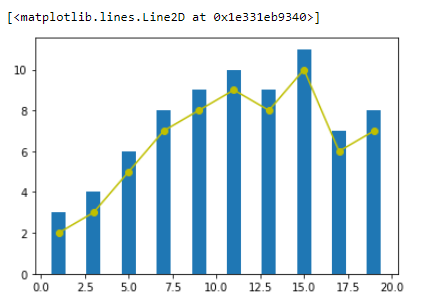# 图形位置

## figure对象

``````# 生成数据
x = np.linspace(0, 10, 20)
y = x * x + 2

# 新建图形对象
fig = plt.figure()
# 控制画布的左, 下, 宽度, 高度
axes = fig.add_axes([0.5, 0.5, 0.8, 0.8])
axes.plot(x, y, 'r')
``````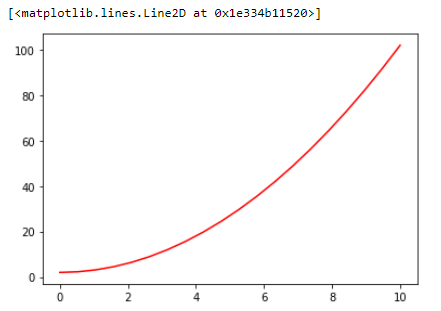Matplotlib 的 API 设计的非常符合常理，在这里，`figure` 相当于绘画用的画板，而 `axes` 则相当于铺在画板上的画布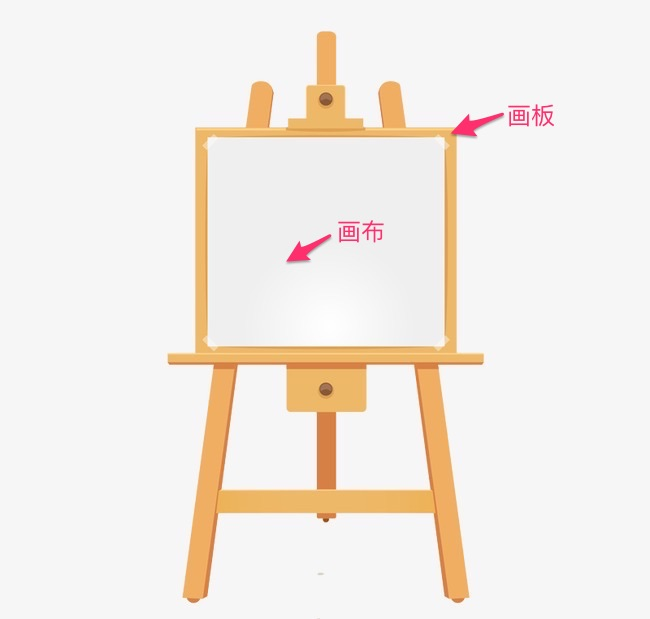``````# 新建画板
fig = plt.figure()

# 大画布
# 小画布

# 大画布
axes1.plot(x,y,'r')
# 小画布
axes2.plot(x,y,'g')
``````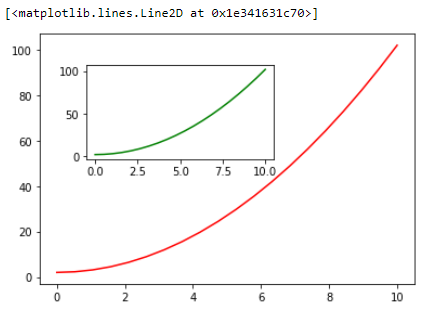## subplots对象

``````flg,axes = plt.subplots()
axes.plot(x,y,'r')
``````

``````# 子图为 1 行，2 列
flg,axes = plt.subplots(nrows=1,ncols=2)
for ax in axes:
ax.plot(x,y,'r')
``````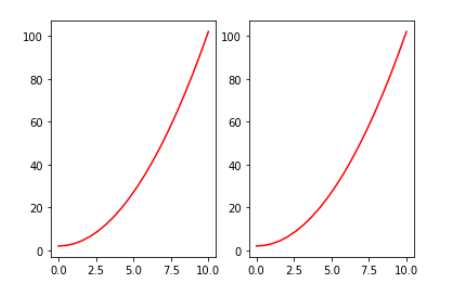``````x = [1,2,3]
y = [1,2,3]

flg,axes = plt.subplots(nrows=1,ncols=2)
axes.plot(x,y,'r')
axes.plot(x[::-1],y,'r')
``````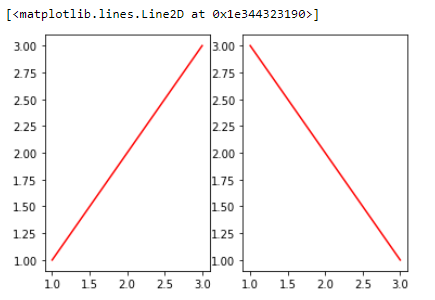``````fig, axes = plt.subplots(
# 通过 figsize 调节尺寸, dpi 调节显示精度
figsize=(16, 9), dpi=50
)

axes.plot(x, y, 'r')
``````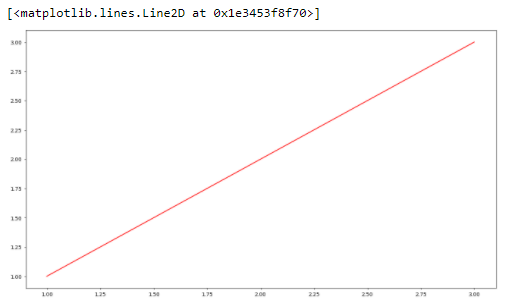# 规范绘图方法

## 添加图标题/图例

``````fig, axes = plt.subplots()

# 横轴名称
axes.set_xlabel('x label')
axes.set_ylabel('y label')

# 图形名称
axes.set_title('title')

axes.plot(x, x**2)
axes.plot(x, x**3)
# 图例
axes.legend(["y = x**2", "y = x**3"], loc=0)
``````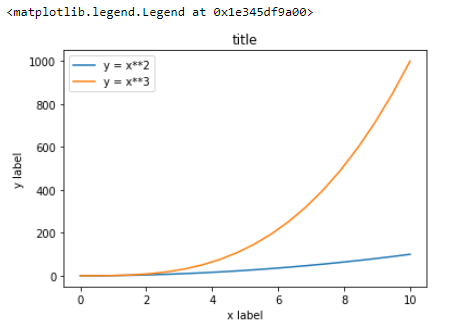## 线型、颜色、透明度

``````fig,axes = plt.subplots()

axes.plot(x, x+1, color="red", alpha=0.5)
axes.plot(x, x+2, color="#1155dd")
axes.plot(x, x+3, color="#15cc55")
``````

``````fig, ax = plt.subplots(figsize=(12, 6))

# 线宽
ax.plot(x, x+1, color="blue", linewidth=0.25)
ax.plot(x, x+2, color="blue", linewidth=0.50)
ax.plot(x, x+3, color="blue", linewidth=1.00)
ax.plot(x, x+4, color="blue", linewidth=2.00)

# 虚线类型
ax.plot(x, x+5, color="red", lw=2, linestyle='-')
ax.plot(x, x+6, color="red", lw=2, ls='-.')
ax.plot(x, x+7, color="red", lw=2, ls=':')

# 虚线交错宽度
line, = ax.plot(x, x+8, color="black", lw=1.50)
line.set_dashes([5, 10, 15, 10])

# 符号
ax.plot(x, x + 9, color="green", lw=2, ls='--', marker='+')
ax.plot(x, x+10, color="green", lw=2, ls='--', marker='o')
ax.plot(x, x+11, color="green", lw=2, ls='--', marker='s')
ax.plot(x, x+12, color="green", lw=2, ls='--', marker='1')

# 符号大小和颜色
ax.plot(x, x+13, color="purple", lw=1, ls='-', marker='o', markersize=2)
ax.plot(x, x+14, color="purple", lw=1, ls='-', marker='o', markersize=4)
ax.plot(x, x+15, color="purple", lw=1, ls='-',
marker='o', markersize=8, markerfacecolor="red")
ax.plot(x, x+16, color="purple", lw=1, ls='-', marker='s', markersize=8,
markerfacecolor="yellow", markeredgewidth=2, markeredgecolor="blue")
``````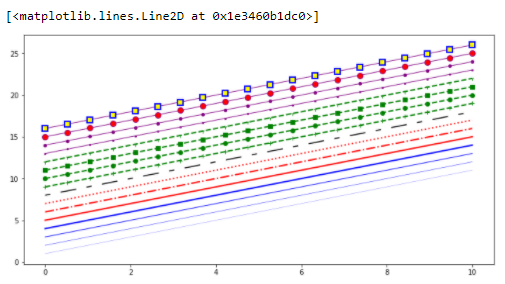## 画布网格、坐标轴范围

``````fig, axes = plt.subplots(1, 2, figsize=(10, 5))

# 显示网格
axes.plot(x, x**2, x, x**3, lw=2)
axes.grid(True)

# 设置坐标轴范围
axes.plot(x, x**2, x, x**3)
axes.set_ylim([0, 60])
axes.set_xlim([2, 5])
``````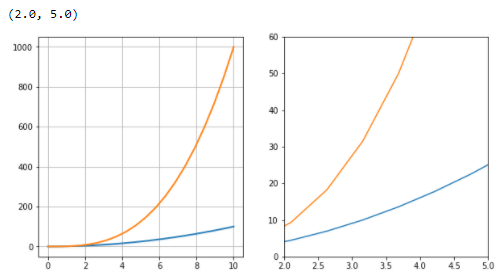``````n = np.array([0, 1, 2, 3, 4, 5])

fig, axes = plt.subplots(1, 4, figsize=(16, 5))

axes.scatter(x, x + 0.25*np.random.randn(len(x)))
axes.set_title("scatter")

axes.step(n, n**2, lw=2)
axes.set_title("step")

axes.bar(n, n**2, align="center", width=0.5, alpha=0.5)
axes.set_title("bar")

axes.fill_between(x, x**2, x**3, color="green", alpha=0.5)
axes.set_title("fill_between")
``````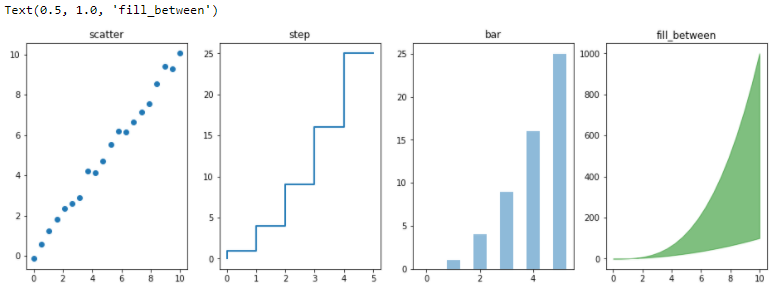# 图形标注方法

Matplotlib 中，文字标注的方法由 `matplotlib.pyplot.text()` 实现

``````fig,axes = plt.subplots()

# 柱形图横坐标
x_bar = [
10,20,30,40,50
]
# 柱形图纵坐标
y_bar = [
0.5,0.6,0.3,0.4,0.8
]

# 绘制柱形图
bars = axes.bar(x_bar,y_bar,color="blue",label=x_bar,width=2)

for i,rect in enumerate(bars):
# 获取柱形图纵坐标
x_text = rect.get_x()
# 获取柱子的高度并增加0.01
y_text = rect.get_height() + 0.01
# 标注文字
plt.text(x_text,y_text,'%.1f' % y_bar[i])
``````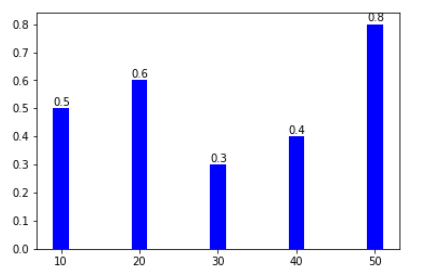``````for i, rect in enumerate(bars):
# 获取柱形图纵坐标
x_text = rect.get_x()
# 获取柱子的高度并增加0.01
y_text = rect.get_height() + 0.01
# 标注文字
plt.text(x_text, y_text, '%.1f' % y_bar[i])

# 增加箭头标注
plt.annotate(
'Min', xy=(32, 0.3), xytext=(36, 0.3),